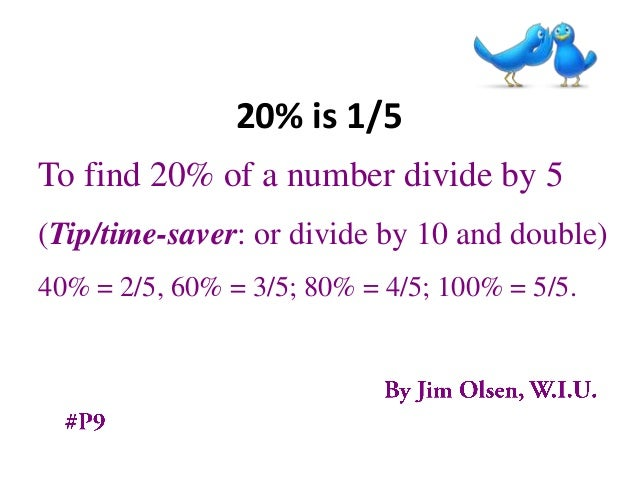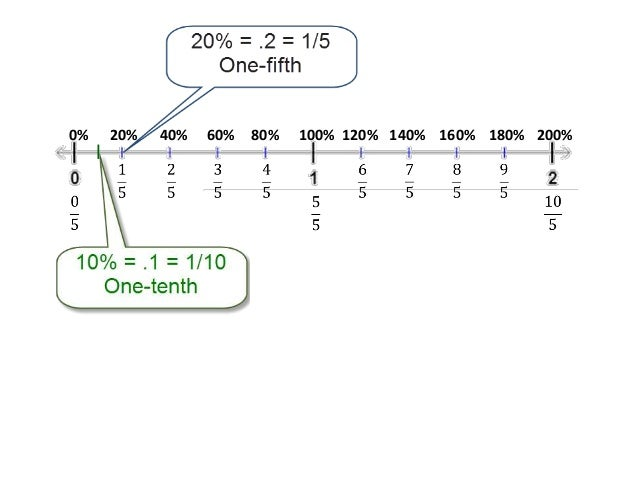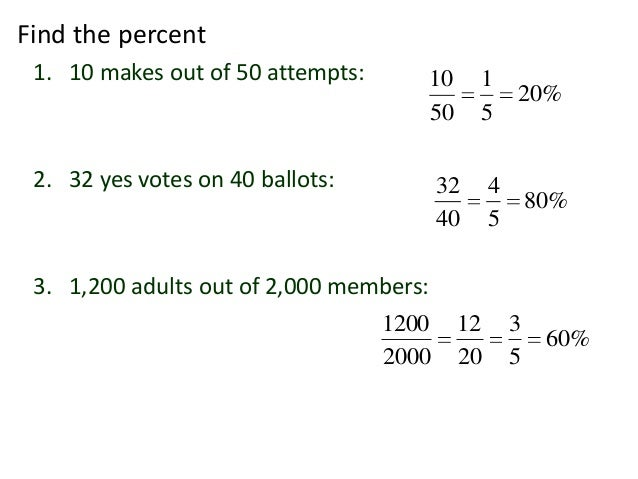Successfully reported this slideshow.Upcoming SlideShare
×

ofUpcoming SlideShare
Finding the Percent in Percent Problems
Next

0

Share

# 20 Percent is One-Fifth

20% is 1/5
To find 20% of a number divide by 5 (Tip/time-saver: or divide by 10 and double) 40% = 2/5 etc.
Learnist Board: http://bit.ly/13AGhZq
#P9

See all

### Related Audiobooks

#### Free with a 30 day trial from Scribd

See all
• Be the first to like this

### 20 Percent is One-Fifth

1. 1. 20% is 1/5 To find 20% of a number divide by 5 (Tip/time-saver: or divide by 10 and double) 40% = 2/5, 60% = 3/5; 80% = 4/5; 100% = 5/5.
2. 2. 0% 20% 40% 60% 80% 100% 120% 140% 160% 180% 200%
3. 3. Find the percent 1. 10 makes out of 50 attempts: 10 1 20% 50 5 2. 32 yes votes on 40 ballots: 32 4 80% 40 5 3. 1,200 adults out of 2,000 members: 1200 12 3 60% 2000 20 5
4. 4. If the number is easily divisible by 5: To find 20% of a number divide by 5 Examples: 20% of 30 is 6 20% of 45 is 9
5. 5. If the number is not easily divisible by 5: Divide by 10 and double Examples: 20% of 31 is 6.2 20% of 230 is 46
6. 6. Recall the strategy: To find A% of B You can change the percent to a fraction, Find B divided by the bottom, then Multiply by the top. Find 60% of 35 3 45 60% of 45 45 3 9 3 27 5 5
7. 7. Examples: 1. If the total revenue is \$500 and 40% is profit, how much is profit? 2 40% of 500 500 \$200 5 2. If to make 30 tons cement mixture we 60% sand, how much sand do we need? 60% of 30 18 tons 3. This year’s sales are 120% of last year’s sales. If last year we sold \$4,500, what are sales this year? 6 4500 120% of 4500 = of 4500 6 900 6 \$5, 400 5 5 4. If commission is 20% and the sale was \$2,340, what is the commission? 2340 234 \$468
8. 8. Closing Notes  Remember 

Total views

12,799

On Slideshare

0

From embeds

0

Number of embeds

128

2

Shares

0